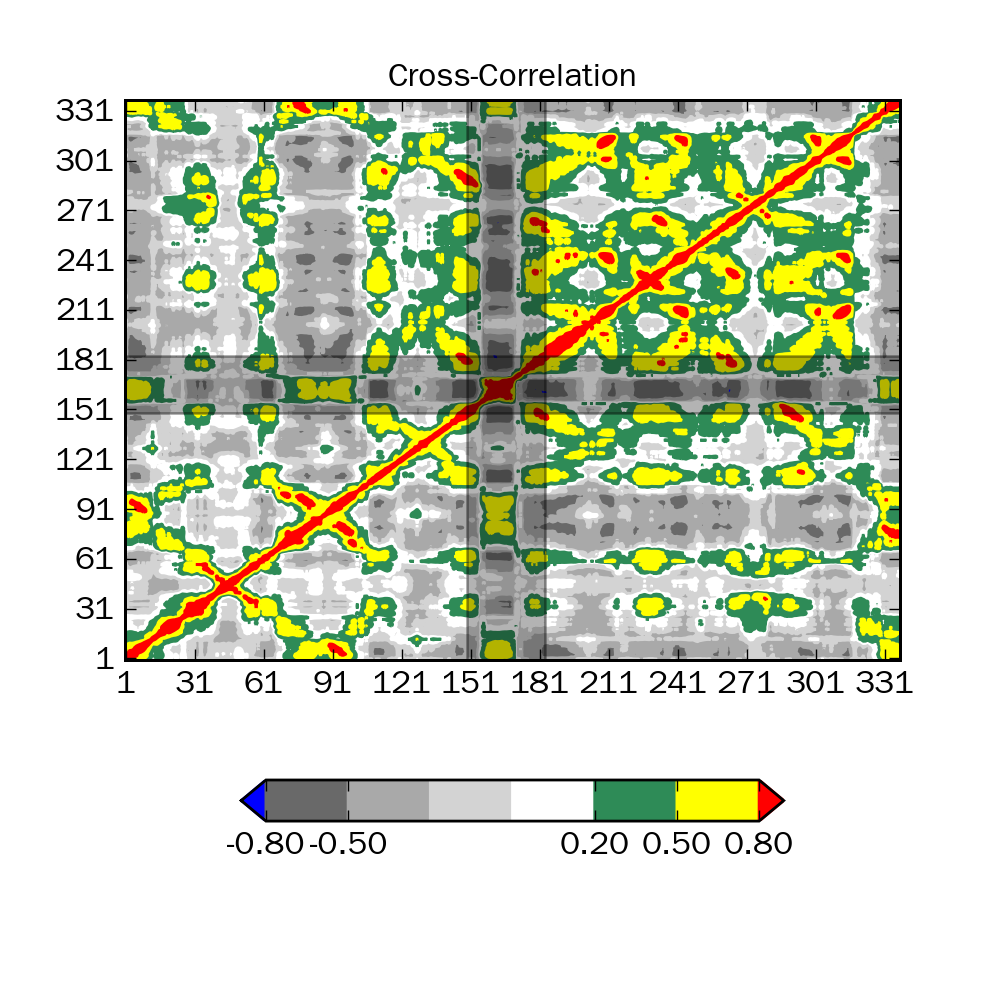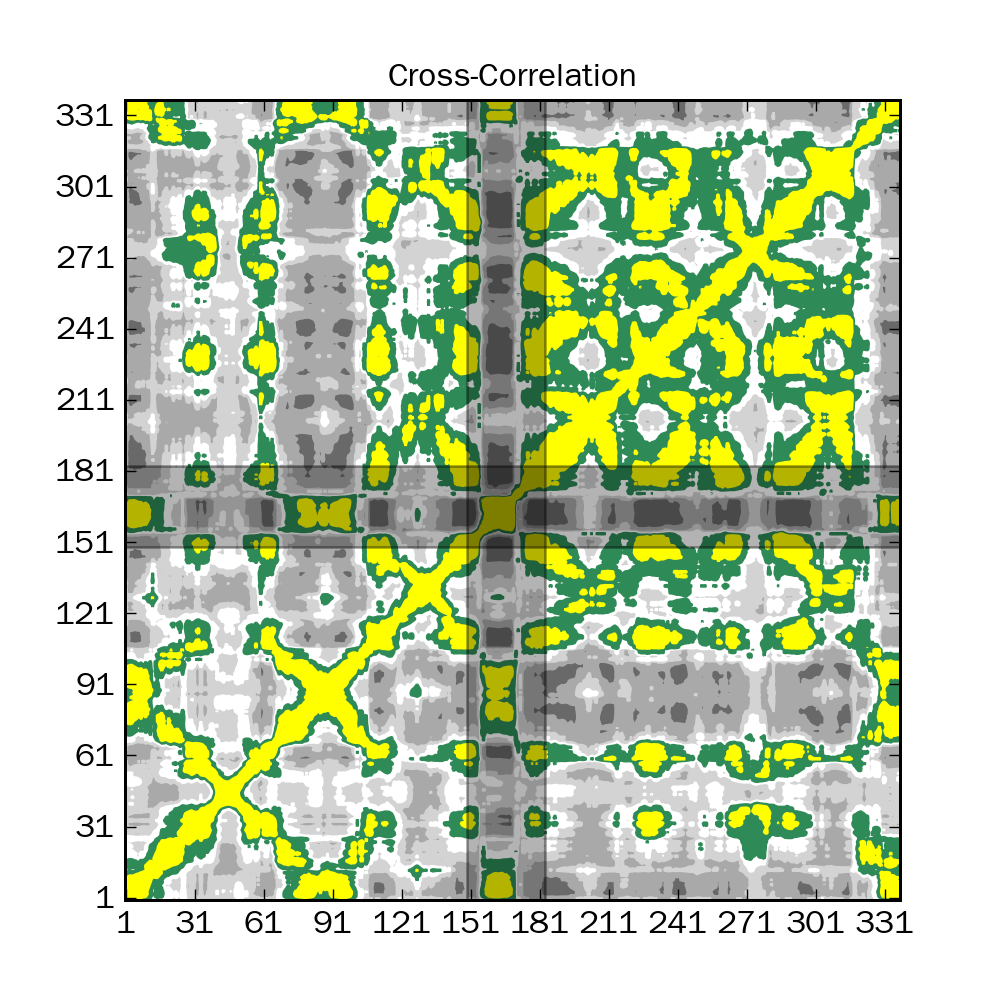# contouf and colorbar

Friends,
I wrote a small program to plot a matrix using contourf function. The code is pasted below. The image that is created is attached (1.png). Now if i make the same figure, just by omitting the colorbar by commenting the line, then i see that the contourf output is different (2.png). Why this difference ? Whether i include colorbar or not, the contourf should create similar plot right ?. Am i wrong something wrong in the code. Kindly help me to understand the problem.
CODE
#!/usr/bin/env python

# USAGE: python script matrix-file

import matplotlib.pyplot as plt
import matplotlib.colors as clr
import numpy as np
from sys import argv
import matplotlib as mpl
# Assignments
mpl.rcParams[‘font.family’]=‘bold’
mpl.rcParams[‘font.size’]=12.0
FIG=plt.figure(figsize=(5,5))
ax1.set_title(‘Cross-Correlation’,size=12,fontweight=‘bold’)
ax1.set_xlim(auto=True)
ax1.set_ylim(auto=True)
ax1.set_xticks(range(1,len(inmat),30))
ax1.set_yticks(range(1,len(inmat),30))
ax1.axvspan(149,183,facecolor=‘black’,alpha=0.3)
ax1.axhspan(149,183,facecolor=‘black’,alpha=0.3)
lev=[-0.8,-0.5,-0.2,0,0.2,0.5,0.8]
col=[’#696969’,’#a9a9a9’,’#d3d3d3’,’#ffffff’,’#2e8b57’,’#ffff00’]
# Define color map
cmap=clr.ListedColormap(col)
norm=clr.BoundaryNorm(lev, cmap.N)
# Make the contourf
CNF=ax1.contourf(inmat,cmap=cmap,norm=norm,levels=lev,origin=‘lower’,extend=‘both’)
CNF.cmap.set_under(‘blue’)
CNF.cmap.set_over(‘red’)
# set up colorbar
#plt.colorbar(CNF,ticks=[-0.8,-0.5,0.2,0.5,0.8],shrink=0.7,format="%.2f",orientation=‘horizontal’,aspect=12)
plt.savefig(‘img.png’,dpi=200)Bala: The shape of the plot changes, because colorbar adjusts the axes to make room for itself. Otherwise the plots look identical. Am I missing something?

-Jeff

···

On 10/14/10 5:21 AM, Bala subramanian wrote:

Friends,
I wrote a small program to plot a matrix using contourf function. The code is pasted below. The image that is created is attached (1.png). Now if i make the same figure, just by omitting the colorbar by commenting the line, then i see that the contourf output is different (2.png). Why this difference ? Whether i include colorbar or not, the contourf should create similar plot right ?. Am i wrong something wrong in the code. Kindly help me to understand the problem.

Jeff, Bala:

I see what is wrong… the blues and reds are missing if Bala takes out the colorbar call. This is because the calls to cmap.set_under() and cmap.set_over() are being done after the call to contourf(). Somehow, if colorbar() is called, then the data in the colormap gets updated for the process of rendering the colorbar, but it does not happen otherwise.

A lot of stuff in the colormaps are designed around the idea of deferred processing to save memory and processing time. I am not sure if this is a bug or not, but I would have expected that the call to show() would properly updated the contourf data, irrespective of the call to colorbar or not. I would be curious to see what would happen if set_under and set_over were called after the call to colorbar.

Bala, in case you haven’t noticed yet, a proper workaround would be calling set_under and set_over before calling contourf().

Ben Root

···

On Thu, Oct 14, 2010 at 7:58 AM, Jeff Whitaker <jswhit@…146…> wrote:

On 10/14/10 5:21 AM, Bala subramanian wrote:

Friends,

I wrote a small program to plot a matrix using contourf function. The

code is pasted below. The image that is created is attached (1.png).

Now if i make the same figure, just by omitting the colorbar by

commenting the line, then i see that the contourf output is different

(2.png). Why this difference ? Whether i include colorbar or not, the

contourf should create similar plot right ?. Am i wrong something

wrong in the code. Kindly help me to understand the problem.

Bala: The shape of the plot changes, because colorbar adjusts the axes

to make room for itself. Otherwise the plots look identical. Am I

missing something?

-Jeff